College Chemistry : Colligative Properties

Example Questions

Example Question #51 : College Chemistry

Which of the following solutions would be expected to have the highest osmotic pressure?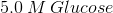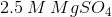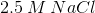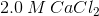Explanation:

In this question, we're asked to identify an answer choice that would be expected to give us a solution with the greatest osmotic pressure. Remember that osmotic pressure is proportional to the total number of dissolved solute particles in solution, regardless of the identity of those solute particles.

When looking at the answer choices, we need to keep in mind two things. First, we need to recognize the numerical value given for the concentration of the compound given. Secondly, we need to identify if the compound shown is capable of dissociating in solution to give rise to even more solute particles. This is important, as it would affect the osmotic pressure.would be expected to have the largest osmotic pressure because, in total, this would be a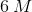solution after dissociation occurs.

Example Question #52 : College Chemistry

Which of the following is not a colligative property?

Electronegativity

Osmotic pressure

Vapor pressure depression

Freezing point depression

Electronegativity

Explanation:

Colligative properties are properties of solutions which depend on the number of dissolved particles in solution. The four main colligative properties are:

1) Freezing point depression: The presence of a solute lowers the freezing point of a solution as compared to that of the pure solvent.

2) Boiling point elevation: The presence of a solute increases the boiling point of a solution as compared to that of the pure solvent.

3) Vapor pressure depression: The vapor pressure of a pure solvent is greater than that of a solution containing a non-volatile liquid. The lowering of vapor pressure leads to boiling point elevation.

4) Osmotic pressure: The osmotic pressure of a solution is the pressure difference between the solution and pure solvent when the two are in equilibrium across a semipermeable membrane. Because it depends on the concentration of solute particles in solution, it is a colligative property.

Electronegativity is not a property of solutions reliant on the number of dissolved particles, but a property of atoms themselves.

Example Question #53 : College Chemistry

Which of the following aqueous solutions would be expected to have the greatest increase in boiling point?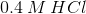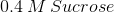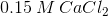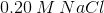Explanation:

This question is asking us to identify a solution that increases the boiling point of water by the greatest amount.

To answer this, we need to understand the concept of colligative properties. When a solute dissolves in a solvent such as water, various physical properties are affected. The four colligative properties that change as a result of the addition of solute are freezing point, boiling point, vapor pressure, and osmotic pressure.

With regards to boiling point, as more solute is added to a solution, the boiling point increases. This is due to the fact that addition of solute makes it more difficult for the solute molecules to gain enough kinetic energy at the solution's surface to escape as a gas.

Furthermore, the identity of the solute does not matter. Thus, we need to look only at the number of dissolved solute particles rather than their identity. A compound such as sucrose will not dissociate in solution, which means that the osmotic pressure of the solution is the same as the concentration of sucrose.

Compounds that can dissociate into two or more particles will increase the osmolarity of the solution further. In this case,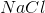will double the stated osmolarity.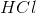, on the other hand, will dissociate completely because it is a strong acid, however the protons will not contribute to the osmolarity.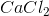is able to dissociate into three equivalents of particles in solution. Thus, its initial concentration will be tripled, which gives it the highest osmolarity of any of the choices shown and will thus increase the boiling point by the greatest amount.

All College Chemistry Resources